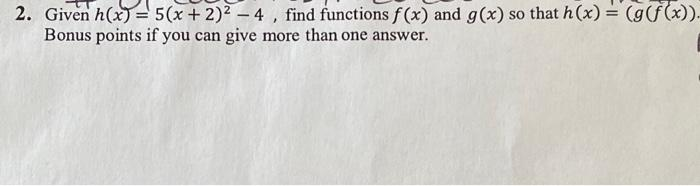Home / Expert Answers / Algebra / 2-given-h-x-5-x-2-2-4-find-functions-f-x-and-g-x-so-that-h-x-g-f-pa869

# (Solved): 2. Given \( h(x)=5(x+2)^{2}-4 \), find functions \( f(x) \) and \( g(x) \) so that \( h(x)=(g(f ...

????2. Given \( h(x)=5(x+2)^{2}-4 \), find functions \( f(x) \) and \( g(x) \) so that \( h(x)=(g(f(x)) \). Bonus points if you can give more than one answer.

We have an Answer from Expert